Publicité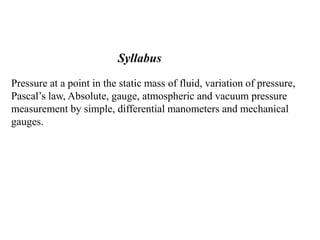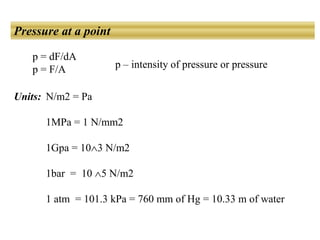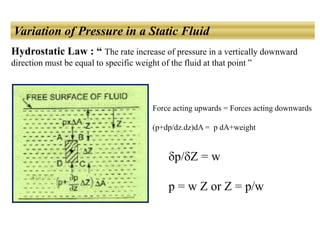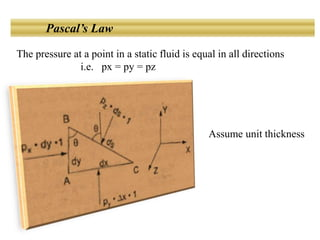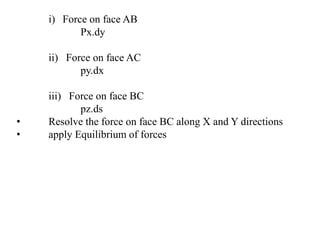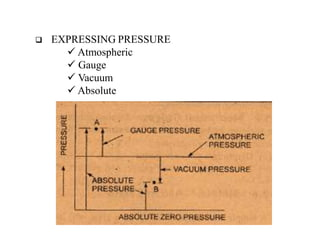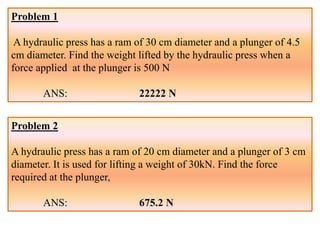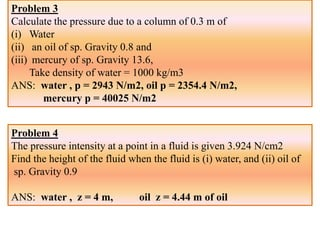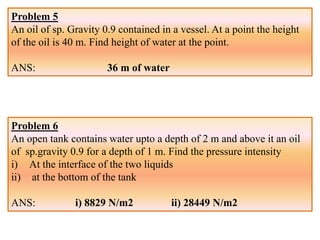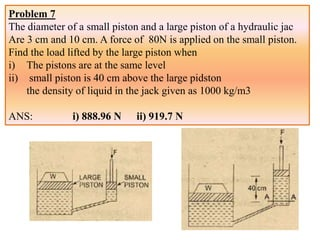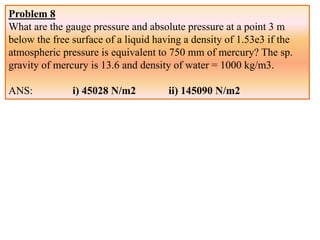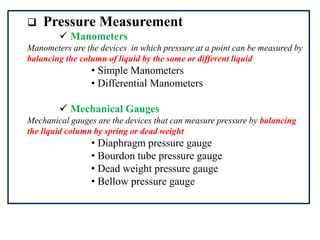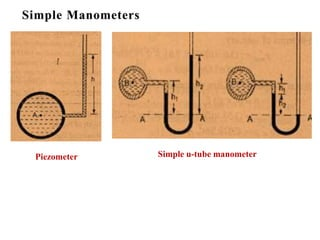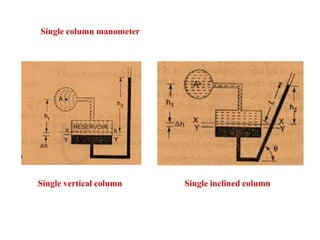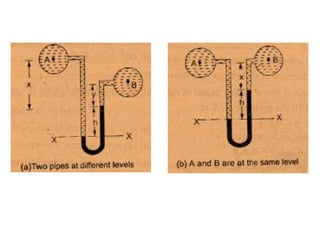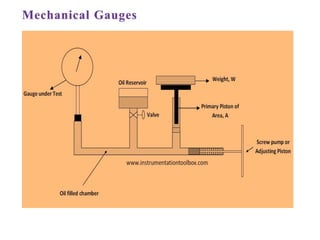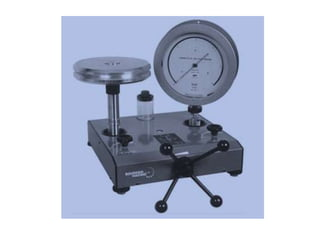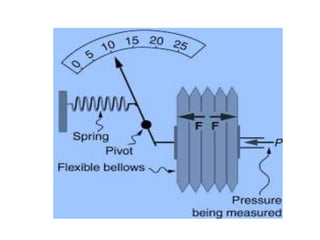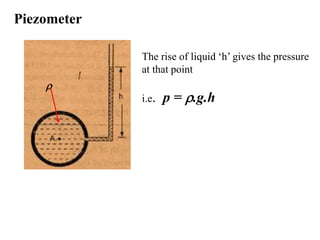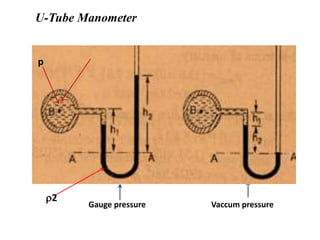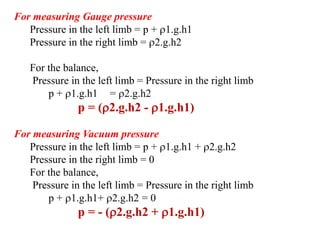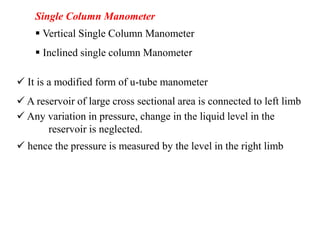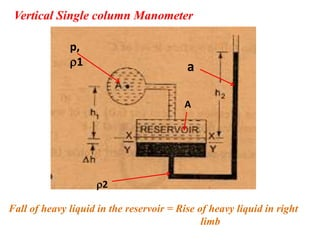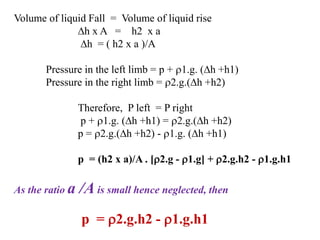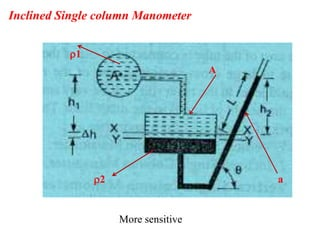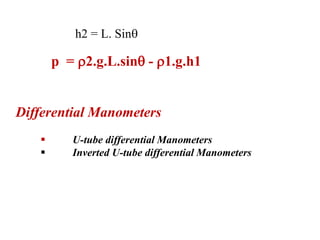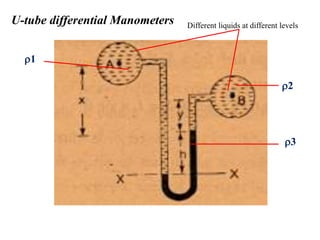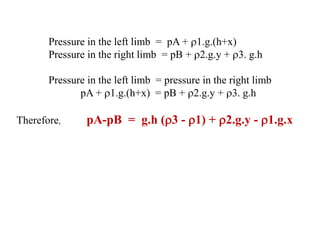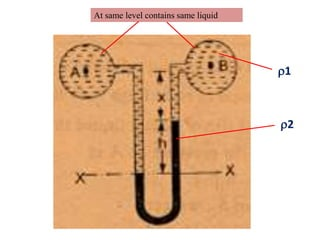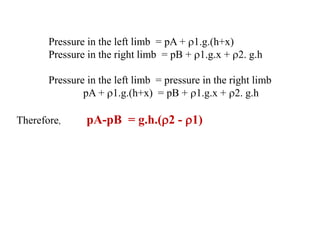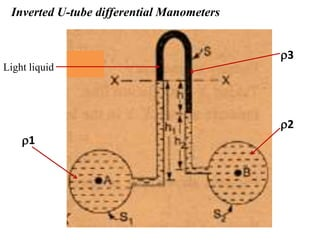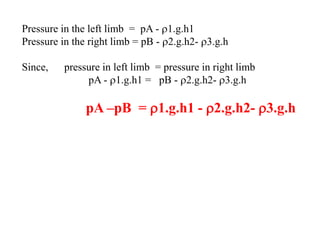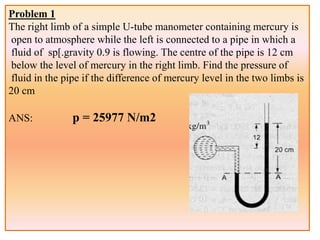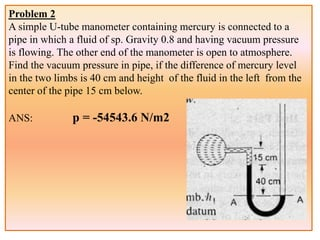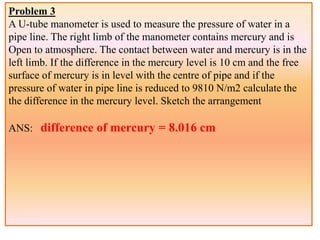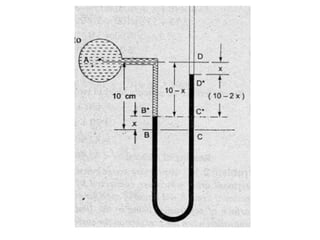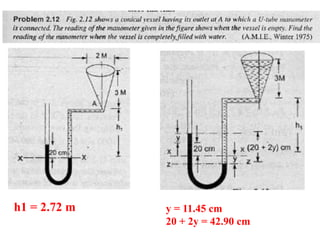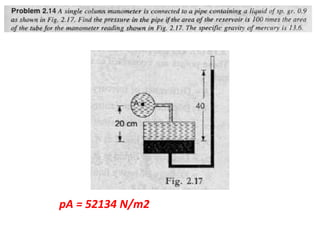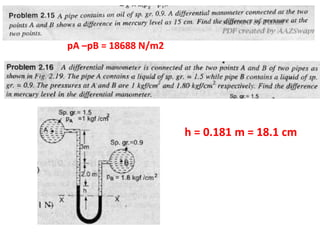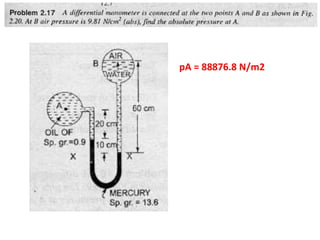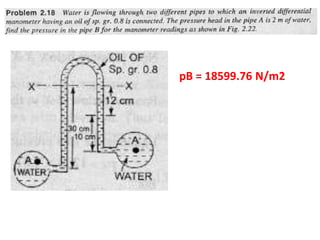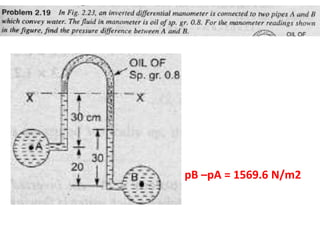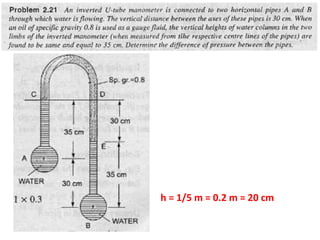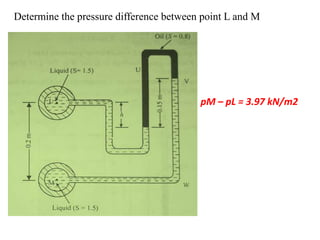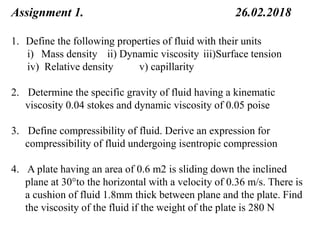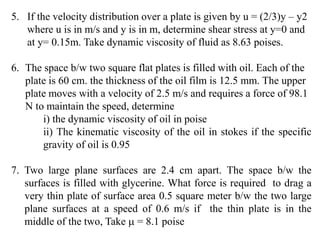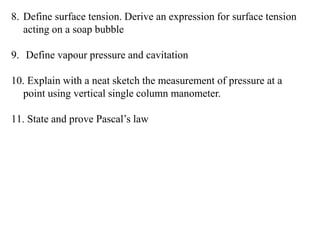1 sur 47
Publicité

### Pressure and its Measurement

1. Pressure at a point in the static mass of fluid, variation of pressure, Pascal’s law, Absolute, gauge, atmospheric and vacuum pressure measurement by simple, differential manometers and mechanical gauges. Syllabus
2. Pressure at a point p = dF/dA p = F/A p – intensity of pressure or pressure Units: N/m2 = Pa 1MPa = 1 N/mm2 1Gpa = 103 N/m2 1bar = 10 5 N/m2 1 atm = 101.3 kPa = 760 mm of Hg = 10.33 m of water
3. Variation of Pressure in a Static Fluid Hydrostatic Law : “ The rate increase of pressure in a vertically downward direction must be equal to specific weight of the fluid at that point ” p/Z = w p = w Z or Z = p/w Force acting upwards = Forces acting downwards (p+dp/dz.dz)dA = p dA+weight
4. Pascal’s Law The pressure at a point in a static fluid is equal in all directions i.e. px = py = pz Assume unit thickness
5. i) Force on face AB Px.dy ii) Force on face AC py.dx iii) Force on face BC pz.ds • Resolve the force on face BC along X and Y directions • apply Equilibrium of forces
6.  EXPRESSING PRESSURE  Atmospheric  Gauge  Vacuum  Absolute
7. Problem 1 A hydraulic press has a ram of 30 cm diameter and a plunger of 4.5 cm diameter. Find the weight lifted by the hydraulic press when a force applied at the plunger is 500 N ANS: 22222 N Problem 2 A hydraulic press has a ram of 20 cm diameter and a plunger of 3 cm diameter. It is used for lifting a weight of 30kN. Find the force required at the plunger, ANS: 675.2 N
8. Problem 3 Calculate the pressure due to a column of 0.3 m of (i) Water (ii) an oil of sp. Gravity 0.8 and (iii) mercury of sp. Gravity 13.6, Take density of water = 1000 kg/m3 ANS: water , p = 2943 N/m2, oil p = 2354.4 N/m2, mercury p = 40025 N/m2 Problem 4 The pressure intensity at a point in a fluid is given 3.924 N/cm2 Find the height of the fluid when the fluid is (i) water, and (ii) oil of sp. Gravity 0.9 ANS: water , z = 4 m, oil z = 4.44 m of oil
9. Problem 5 An oil of sp. Gravity 0.9 contained in a vessel. At a point the height of the oil is 40 m. Find height of water at the point. ANS: 36 m of water Problem 6 An open tank contains water upto a depth of 2 m and above it an oil of sp.gravity 0.9 for a depth of 1 m. Find the pressure intensity i) At the interface of the two liquids ii) at the bottom of the tank ANS: i) 8829 N/m2 ii) 28449 N/m2
10. Problem 7 The diameter of a small piston and a large piston of a hydraulic jac Are 3 cm and 10 cm. A force of 80N is applied on the small piston. Find the load lifted by the large piston when i) The pistons are at the same level ii) small piston is 40 cm above the large pidston the density of liquid in the jack given as 1000 kg/m3 ANS: i) 888.96 N ii) 919.7 N
11. Problem 8 What are the gauge pressure and absolute pressure at a point 3 m below the free surface of a liquid having a density of 1.53e3 if the atmospheric pressure is equivalent to 750 mm of mercury? The sp. gravity of mercury is 13.6 and density of water = 1000 kg/m3. ANS: i) 45028 N/m2 ii) 145090 N/m2
12.  Pressure Measurement  Manometers Manometers are the devices in which pressure at a point can be measured by balancing the column of liquid by the same or different liquid • Simple Manometers • Differential Manometers  Mechanical Gauges Mechanical gauges are the devices that can measure pressure by balancing the liquid column by spring or dead weight • Diaphragm pressure gauge • Bourdon tube pressure gauge • Dead weight pressure gauge • Bellow pressure gauge
13. Piezometer Simple u-tube manometer
14. Single column manometer Single vertical column Single inclined column
15. Piezometer The rise of liquid ‘h’ gives the pressure at that point i.e. p = .g.h 
16. U-Tube Manometer 1 2 Gauge pressure Vaccum pressure p
17. For measuring Gauge pressure Pressure in the left limb = p + 1.g.h1 Pressure in the right limb = 2.g.h2 For the balance, Pressure in the left limb = Pressure in the right limb p + 1.g.h1 = 2.g.h2 p = (2.g.h2 - 1.g.h1) For measuring Vacuum pressure Pressure in the left limb = p + 1.g.h1 + 2.g.h2 Pressure in the right limb = 0 For the balance, Pressure in the left limb = Pressure in the right limb p + 1.g.h1+ 2.g.h2 = 0 p = - (2.g.h2 + 1.g.h1)
18. Single Column Manometer  Vertical Single Column Manometer  Inclined single column Manometer  It is a modified form of u-tube manometer  A reservoir of large cross sectional area is connected to left limb  Any variation in pressure, change in the liquid level in the reservoir is neglected.  hence the pressure is measured by the level in the right limb
19. Vertical Single column Manometer p, 1 2 A a Fall of heavy liquid in the reservoir = Rise of heavy liquid in right limb
20. Volume of liquid Fall = Volume of liquid rise h x A = h2 x a h = ( h2 x a )/A Pressure in the left limb = p + 1.g. (h +h1) Pressure in the right limb = 2.g.(h +h2) Therefore, P left = P right p + 1.g. (h +h1) = 2.g.(h +h2) p = 2.g.(h +h2) - 1.g. (h +h1) p = (h2 x a)/A . [2.g - 1.g] + 2.g.h2 - 1.g.h1 As the ratio a /A is small hence neglected, then p = 2.g.h2 - 1.g.h1
21. Inclined Single column Manometer More sensitive 1 2 A a
22. p = 2.g.L.sin - 1.g.h1 h2 = L. Sin Differential Manometers  U-tube differential Manometers  Inverted U-tube differential Manometers
23. U-tube differential Manometers Different liquids at different levels 1 2 3
24. Pressure in the left limb = pA + 1.g.(h+x) Pressure in the right limb = pB + 2.g.y + 3. g.h Pressure in the left limb = pressure in the right limb pA + 1.g.(h+x) = pB + 2.g.y + 3. g.h Therefore, pA-pB = g.h (3 - 1) + 2.g.y - 1.g.x
25. At same level contains same liquid 1 2
26. Pressure in the left limb = pA + 1.g.(h+x) Pressure in the right limb = pB + 1.g.x + 2. g.h Pressure in the left limb = pressure in the right limb pA + 1.g.(h+x) = pB + 1.g.x + 2. g.h Therefore, pA-pB = g.h.(2 - 1)
27. Inverted U-tube differential Manometers 1 2 3 Light liquid
28. Pressure in the left limb = pA - 1.g.h1 Pressure in the right limb = pB - 2.g.h2- 3.g.h Since, pressure in left limb = pressure in right limb pA - 1.g.h1 = pB - 2.g.h2- 3.g.h pA –pB = 1.g.h1 - 2.g.h2- 3.g.h
29. Problem 1 The right limb of a simple U-tube manometer containing mercury is open to atmosphere while the left is connected to a pipe in which a fluid of sp[.gravity 0.9 is flowing. The centre of the pipe is 12 cm below the level of mercury in the right limb. Find the pressure of fluid in the pipe if the difference of mercury level in the two limbs is 20 cm ANS: p = 25977 N/m2
30. Problem 2 A simple U-tube manometer containing mercury is connected to a pipe in which a fluid of sp. Gravity 0.8 and having vacuum pressure is flowing. The other end of the manometer is open to atmosphere. Find the vacuum pressure in pipe, if the difference of mercury level in the two limbs is 40 cm and height of the fluid in the left from the center of the pipe 15 cm below. ANS: p = -54543.6 N/m2
31. Problem 3 A U-tube manometer is used to measure the pressure of water in a pipe line. The right limb of the manometer contains mercury and is Open to atmosphere. The contact between water and mercury is in the left limb. If the difference in the mercury level is 10 cm and the free surface of mercury is in level with the centre of pipe and if the pressure of water in pipe line is reduced to 9810 N/m2 calculate the the difference in the mercury level. Sketch the arrangement ANS: difference of mercury = 8.016 cm
32. h1 = 2.72 m y = 11.45 cm 20 + 2y = 42.90 cm
33. pA = 52134 N/m2
34. pA –pB = 18688 N/m2 h = 0.181 m = 18.1 cm
35. pA = 88876.8 N/m2
36. pB = 18599.76 N/m2
37. pB –pA = 1569.6 N/m2
38. h = 1/5 m = 0.2 m = 20 cm
39. Determine the pressure difference between point L and M pM – pL = 3.97 kN/m2
40. Assignment 1. 26.02.2018 1. Define the following properties of fluid with their units i) Mass density ii) Dynamic viscosity iii)Surface tension iv) Relative density v) capillarity 2. Determine the specific gravity of fluid having a kinematic viscosity 0.04 stokes and dynamic viscosity of 0.05 poise 3. Define compressibility of fluid. Derive an expression for compressibility of fluid undergoing isentropic compression 4. A plate having an area of 0.6 m2 is sliding down the inclined plane at 30to the horizontal with a velocity of 0.36 m/s. There is a cushion of fluid 1.8mm thick between plane and the plate. Find the viscosity of the fluid if the weight of the plate is 280 N
41. 5. If the velocity distribution over a plate is given by u = (2/3)y – y2 where u is in m/s and y is in m, determine shear stress at y=0 and at y= 0.15m. Take dynamic viscosity of fluid as 8.63 poises. 6. The space b/w two square flat plates is filled with oil. Each of the plate is 60 cm. the thickness of the oil film is 12.5 mm. The upper plate moves with a velocity of 2.5 m/s and requires a force of 98.1 N to maintain the speed, determine i) the dynamic viscosity of oil in poise ii) The kinematic viscosity of the oil in stokes if the specific gravity of oil is 0.95 7. Two large plane surfaces are 2.4 cm apart. The space b/w the surfaces is filled with glycerine. What force is required to drag a very thin plate of surface area 0.5 square meter b/w the two large plane surfaces at a speed of 0.6 m/s if the thin plate is in the middle of the two, Take  = 8.1 poise
42. 8. Define surface tension. Derive an expression for surface tension acting on a soap bubble 9. Define vapour pressure and cavitation 10. Explain with a neat sketch the measurement of pressure at a point using vertical single column manometer. 11. State and prove Pascal’s law
Publicité